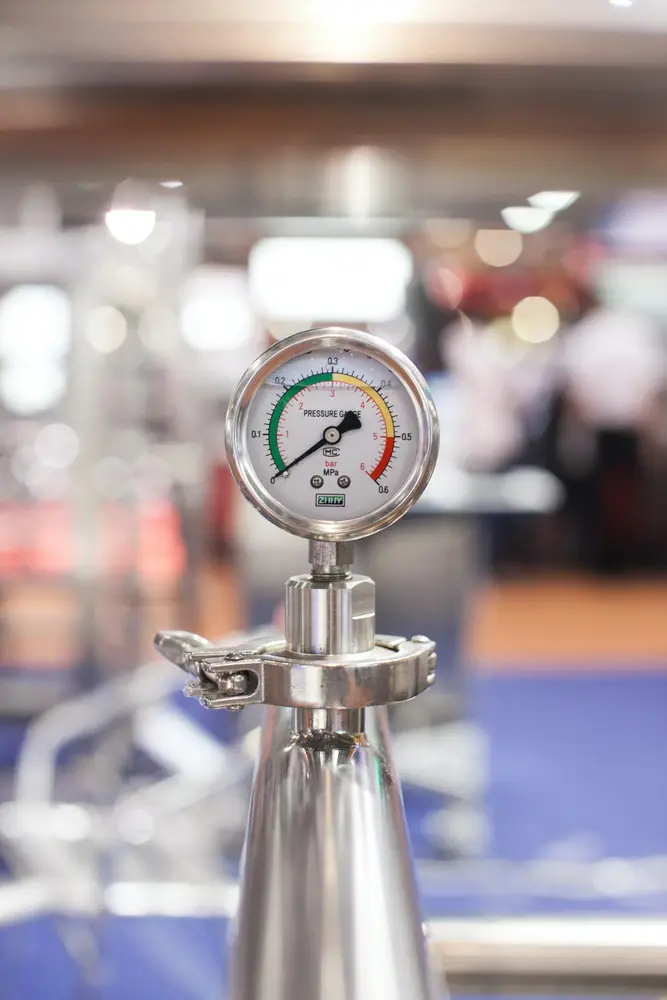# Acceleration

• Acceleration is the rate of change in velocity of an object.
• The most common unit of acceleration is m/s/s which can also be written as m/s2 or ms-2. This means the rate of change in velocity per second.
• For example if an object increased its velocity by 2 metres every second then its acceleration would be 2m/s/s.
• Acceleration = change in velocity / time taken for change.
• Therefore if an object travels from stationary to 5 m/s in 2.5 seconds then its acceleration = 5/2.5 = 2m/s/s.
• Uniform acceleration is just the name given to a constant acceleration.
Forces and motion

#### Forces

##### Forces

Forces and motion

#### Forumulae

##### Forumulae

Forces and motion

#### Hooke's Law

##### Hooke's Law

Forces and motion

#### Momentum

##### Momentum

Forces and motion

#### Pressure

##### Pressure

Forces and motion